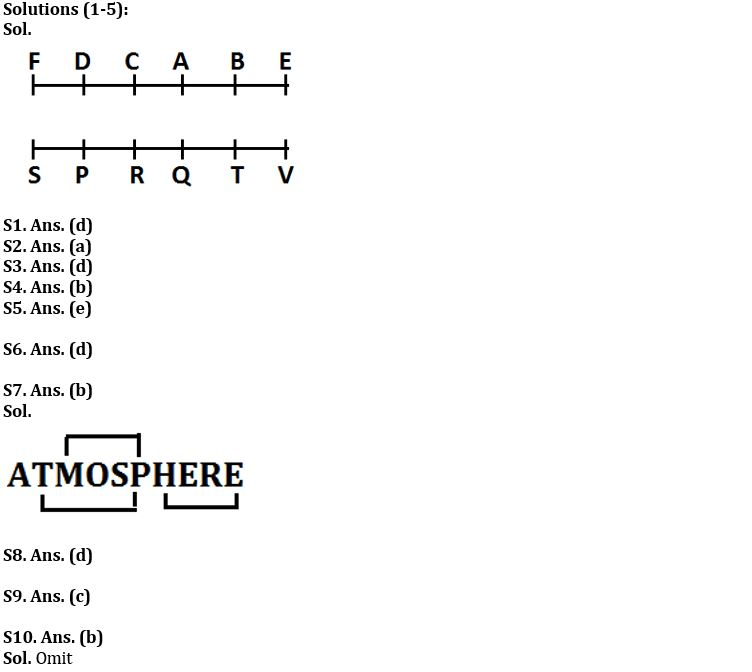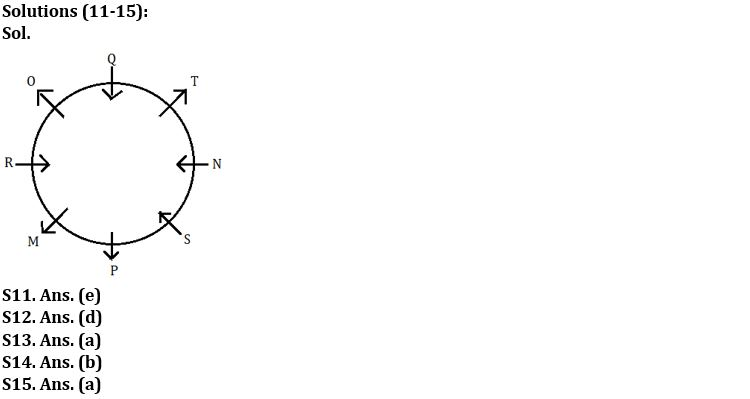Latest Banking jobs   »

# Reasoning Ability Quiz For IBPS Clerk/PO Prelims 2022- 30th August

Directions (1-5): Study the following information carefully and answer the questions given below:
Twelve people are sitting in two parallel rows containing six people each, in such a way that there is an equal distance between adjacent persons. In row–1 A, B, C, D, E and F are seated and all of them are facing south. In row-2 P, Q, R, S, T and V are seated and all of them are facing north. Therefore, in the given seating arrangement each member seated in a row faces another member of the other row.
P sits third to the left of T. Neither P nor T sits at an extreme end of the line. A sits second to the right of E. Neither A nor E faces T or P. A does not sit at an extreme end. R does not face A and R does not sit at an extreme end of the line. Only one person sits between F and C. Neither F nor C faces T. C does not sit at the extreme end. Only one person sits between V and Q. F is not an immediate neighbour of B. A does not face V.

Q1. How many people sit to the right of V?
(a) One
(b) Two
(c) Three
(d) No one
(e) Five

Q2. V is related to B in the same way as Q is related to C. Which of the following is related to P, following the same pattern?
(a) F
(b) A
(c) D
(d) E
(e) None of these

Q3. Which of the following is true regarding V?
(a) V faces A
(b) T is not an immediate neighbour of V.
(c) C faces the one who is second to left of V
(d) V sits at one of the extreme ends of the line
(e) R sits third to the right of V

Q4. Who among the following sit at extreme ends of the rows?
(a) D, F
(b) V, S
(c) Q, S
(d) B, D
(e) None of these

Q5. Who among the following faces B?
(a) V
(b) S
(c) Q
(d) R
(e) T

Q6. Four of the following are alike in a certain way so form a group, which among the following does not belong to that group?
(a) CEHL
(b) PRUY
(c) FHKO
(d) QSUX
(e) JLOS

Q7. How many pairs of letters are there in the word ‘ATMOSPHERE’, each of which as many letters between then in the word have as they have between then in the English alphabet?
(a) One
(b) Three
(c) Two
(d) Four
(e) None

Q8. If in the word GEOGRAPHY, all vowels are replaced by its next letter and all consonant is replaced by its previous letter, then all letters are arranged in alphabetical order from left to right. Then which of the following letter fourth from the right end?
(a) P
(b) B
(c) G
(d) O
(e) F

Q9. If the number ‘53982764’, One is subtracted in odd digit and one is added in even digit of the number then numbers are arranged in ascending order from left to right. Then what is the sum of the digit which is third from the left end and the digit which is fourth from the right end?
(a) 8
(b) 12
(c) 10
(d) 5
(e) None of these

Q10. How many words can be formed from the 1st, 3rd, 6th and 8th letter of a word ‘OPTIMISM’ by using each letter once in the word?
(a) Three
(b) One
(c) None
(d) Two
(e) More than three

Directions (11-15): Study the information carefully and answer the questions given below.
Eight persons M, N, O, P, Q, R, S and T are sitting in a circular table. Some of them face inside and some of them face outside. All information is not necessarily in same order. Not more than two adjacent persons face same direction.
One person sit between P and N. Q sits second to the right of N. Immediate neighbor of N face opposite direction to each other. R sits third to the left of S. R is not immediate neighbor of N and Q. T sits second to the right of O who sits second to the right of M. M sits immediate right of P. T is not immediate neighbor of R. M face outside.

Q11. How many persons are sitting between O and M when counted from right of O?
(a) One
(b) Two
(c) Four
(d) Three
(e) None of these

Q12. Who among the following sits 2nd to right of Q?
(a) O
(b) P
(c) N
(d) R
(e) None of these

Q13. Who among the following sits opposite to T?
(a) M
(b) N
(c) R
(d) O
(e) Q

Q14. Who among the following person sits 3rd to the right person who sits immediate right of M?
(a) Q
(b) S
(c) T
(d) O
(e) None of these

Q15. If Q and P interchange their positions, then who sits third to the left of Q ?
(a) T
(b) Q
(c) R
(d) M
(e) None of these

Solutions#### Congratulations!Download Hindu Review of October 2021: Free PDF